Processing ......FreeComputerBooks.com Links to Free Computer, Mathematics, Technical Books all over the World

Modern Cryptography Volume 1: A Classical Introduction
🌠 Top Free Computer Networking Books - 100% Free or Open Source!
• Title: HModern Cryptography Volume 1: A Classical Introduction
• Author(s) Zhiyong Zheng
• Publisher: Springer; 1st ed. 2022 edition (April 17, 2022); eBook (Creative Commons Licensed)
• Hardcover/Paperback: 370 pages
• eBook: PDF and ePub
• Language: English
• ISBN-10: 9811909229
• ISBN-13: 978-9811909221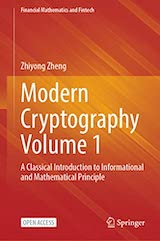Book Description

This open access book systematically explores the statistical characteristics of cryptographic systems, the computational complexity theory of cryptographic algorithms and the mathematical principles behind various encryption and decryption algorithms. The theory stems from technology.

It systematically introduces the information theory, statistical characteristics and computational complexity theory of public key cryptography, focusing on the three main algorithms of public key cryptography, RSA, discrete logarithm and elliptic curve cryptosystem.

• Zhiyong Zheng is Professor and Dean of School of Mathematics, Renmin University of China.
Reviews, Ratings, and Recommendations: Related Book Categories: Read and Download Links:Similar Books:
•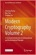Modern Cryptography Volume 2: Post-Quantum Cryptography

This open access book covers the most cutting-edge and hot research topics and fields of post-quantum cryptography. The main purpose of this book is to focus on the computational complexity theory of lattice ciphers.

•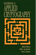Handbook of Applied Cryptography (Alfred J. Menezes, et al)

This book is an incredible achievement. If you want to check what problems there were with a proposed system, determine how the variations on a particular algorithm developed, see what research preceded and followed an idea, go to the Handbook.

•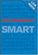Cryptography: An Introduction, 3rd Edition (Nigel Smart)

This book provides the rigorous detail required for advanced cryptographic studies, yet approaches the subject matter in an accessible style in order to gently guide new students through difficult mathematical topics.

•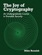The Joy of Cryptography (Mike Rosulek)

The definitive introduction to all major areas of cryptography: message security, key negotiation, and key management, but in a way that is more accessible than what is "traditional" in crypto. All security definitions are written in a "game-based" style.

•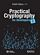Practical Cryptography for Developers (Svetlin Nakov)

A modern practical book about cryptography for developers with code examples, covering core concepts, along with crypto libraries and developer tools, with a lots of code examples in Python and other languages.

•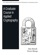A Graduate Course in Applied Cryptography (Dan Boneh, et al)

This book is about mathematical modeling and proofs to show that a particular cryptosystem satisfies the security properties attributed to it: constructing practical cryptosystems for which we can argue security under plausible assumptions.

•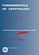Fundamentals of Cryptology: A Professional Reference (H. Tilborg)

This is a thoroughly written wonderful book on cryptography! This excellent book is warmly recommended for mathematicians, computer scientists, for professors and students, and, as a reference book, for researchers in the area of cryptography.

•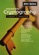Introduction to Modern Cryptography (Mihir Bellare, et al.)

This book introduces the core principles of modern cryptography, with an emphasis on formal definitions, clear assumptions, and rigorous proofs of security. It also presents design principles for widely used stream ciphers and block ciphers.

Book Categories
 :All CategoriesTop Free BooksRecent BooksMiscellaneous BooksComputer EngineeringComputer LanguagesComputer ScienceData Science/DatabasesJava and Java EE (J2EE)Linux and UnixMathematicsMicrosoft and .NETMobile ComputingNetworking and CommunicationsSoftware EngineeringSpecial TopicsWeb Programming
Other Categories